## FORM THREE PHYSICS STUDY NOTES TOPIC TOPIC 7: MEASUREMENT OF THERMAL ENERGY & TOPIC 8: VAPOUR AND HUMIDITY

### FORM THREE PHYSICS STUDY NOTES TOPIC TOPIC 7: MEASUREMENT OF THERMAL ENERGY & TOPIC 8: VAPOUR AND HUMIDITYMonday, April 6, 2020

FORM THREE PHYSICS STUDY NOTES TOPIC TOPIC 7: MEASUREMENT OF THERMAL ENERGY & TOPIC 8: VAPOUR AND HUMIDITY

UNAWEZA JIPATIA NOTES ZETU KWA KUCHANGIA KIASI KIDOGO KABISA:PIGA SIMU:0787237719

## FORM THREE PHYSICS STUDY NOTES TOPIC TOPIC 7: MEASUREMENT OF THERMAL ENERGY & TOPIC 8: VAPOUR AND HUMIDITY

TOPIC TOPIC 7: MEASUREMENT OF THERMAL ENERGY

Heat Capacity
Heat capacity is the amount of heat required to raise the temperature of an object or substance by one degree. The temperature change is the difference between the final temperature ( Tf) and the initial temperature ( Ti).
The Factors which Determine Heat Quality of a Substance
Explain the factors which determine heat quality of a substance
Heat is a form of energy transferred between bodies due to difference in temperature between them. The energy possessed by the body due to its temperature is called the internal thermal energy. The heat content is due to the random motion of the particles that make up the body. The heat content is determined by its mass, temperature change and the specific heat capacity of the substance.
The Heat Capacity
Determine the heat capacity
Heat capacity is the quantity of heat required to raise the temperature of a substance by one degree Celsius.
Heat capacity = mass of the substance X specific heat capacity
Thus H.C = MC
AlsoExample 1
Find the heat capacity of a lump of copper of mass 50kg. The specific heat capacity of copper is 420 J/ Kg ºc.
Data Given
Mass of copper, M= 50kg
The specific heat capacity of copper, C = 420J/KgºC
Required: To calculate heat capacity, H.C.
H.C = MC
= 50Kg x 420J/KgºC
= 21600J
=21KJ
Calculating a quantity of heat
• The quantity of heat required to change the temperature of a body with mass, M Kg by Q degree Celsius is MCQ joules.
• In order to raise the temperature of a body, heat must be supplied to it.
• In order to lower its temperature, heat must be removed from it.
The Heat Equation is therefore written:
Heat Gained or Heat Lost = Mass X specific heat capacity X change in temperature
Change in temperature:
H =MCQ
Where
H=Heat gained / lost
M= Mass of the body
Q= change (Rise or fall) In Temperature of the body.
Example 2
Water of mass 3kg is heated from 26ºc to 96ºC. Find the amount of heat supplied to the water given that the specific heat capacity of water is 4.2 x 103 J / Kg ºc
Data Given
Mass of water, M = 3Kg
Specific Heat capacity, C = 4.2 X 103 j / Kgº C
Initial temperature, Qi= 26 ºC
Final Temperature, Qf = 96ºC
Required
The amount of heat, H
H= MCQ
C = H/MQ
H = MCQ
H = 3Kg x 4.2 x103 (96-26) ºC
C= 882000J= 882KJ
The Specific Heat Capacity
Determine the specific heat capacity
Specific heat capacity is the quantity of heat required to raise the temperature of a unit mass of a substance by one degree Celsius.
The quantity of heat supplied to or taken away from a body depends on:
1. The mass of the body, M
2. The temperature different, ΔT
3. The thermal properties of the body.
Transfer of Heat
Heat energy tends to flow from High temperatures to Low temperatures
If you pick up a warm object, heat energy transfers from the object to your hands and your hands feel warm. If you pick up a cool object, heat energy transfers from hands to the object and your hands feel cold.
Determining specific Heat capacity
• Calorimeter – Is the special instrument or vessel used for measurement of Heat.
• Calorimeter is highly polished metal can usually made of copper or aluminium.
• It is flitted with an insulating cover in which there are two holes.
• Two holes allow a thermometer and a stirrer to be inserted.
• The stirrer is made of the same metal as that of the calorimeter.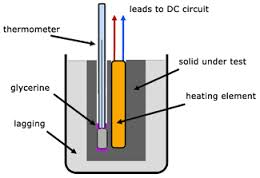Demonstration of the specific Heat capacity of a solid
Determining specific Heat capacity by Method of Calculation.
Heat lost by solid, Hs = Ms x Cs (Qs – Qf)
Heat Gained by Calorimeterand stirrer, Hc = Mc x Cc (Qf – Qi)
Heat Gained by Water, HW = Mw x Cw (Qf – Qi)
But the heat lost by the solid is equal to heat gained by the calorimeter and stirrer plus the heat gained by the water in the calorimeter.
Hs = Hc + Hw
But
Heat gained by a calorimeter and content equal to heat lost by the solid.
Thus Hc + Hl = Hs
Mc Cc (Qf – Qi) + Mi Ci ( Qf-Qi) = Ms Cs ( Qs-Qs)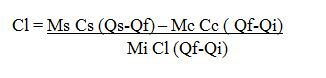Example 3
A piece of metal with a mass of 200g at a temperature of 100ºC is quickly transferred into 50g of water at 20ºC find the final temperature of the system ( specific Heat capacity of water Cw = 4200J/ Kg ºC specific Heat capacity of the metal Cm = 400J/KgºC.
Ms Cs (Qs-Qf) = Mc Cc (Qf-Q) Mm Cw (Qf-Ql)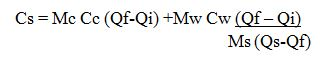Where:
Cs. Is the specific Heat capacity of the solids.
Determining the specific heat capacity of liquid, Cl
By calculation method;
Heat Gained by calorimeter and stirrer
Hc = Mc Cc (Qf – Qi)
Heat Gained by liquid
Hi = ML CL (Qf – Qi)
Heat lost by the solid
Hs = Ms Cs ( Qs-Qf)
Let
Q be the final Temperature of the system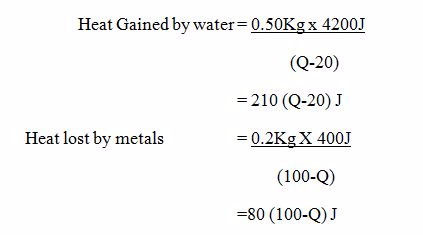If there are no heat Losses to the surroundings, then.
(Heat gained by water) = (Heat lost by metal)
210 (Q-20) = 80(100 – Q)
21(Q-20) = 8 (100-Q)
21Q – 420 = 800 – 8Q
21Q +8Q = (800+420)
29Q = 1220
Q = (1220/29)
Q = 42.1ºC

### TOPIC 8: VAPOUR AND HUMIDITY

Vapour
The Process of Evaporation of Liquid
Explain the process of evaporation of liquid
Vapours
• These are molecules which escape into the atmosphere after liquids are heated.
• When a liquid is heated strongly then molecules tends to escape ( those molecules are called vapour).
• Most liquids evaporates at any temperature however liquids may vary in the rate at which they evaporate at ordinary temperature.
• Alcohol and ether evaporate rapidly but lubricating oil and mercury hardly evaporate.
• Evaporation of a liquids result in the formation of vapour.
Factors Affecting Evaporation of a Liquid
Identify factors affecting evaporation of a liquid
There are several factors which affect evaporation of liquids when heated which include the following:
1. Nature of the liquid: Normally liquids evaporation differs depending on the nature of liquid. Example; Volatile liquids evaporate faster than non-volatile liquids, which evaporate slowly. Alcohol evaporates faster than other liquids like water. The boiling point of alcohol is 780C while that of water is 100oC.
2. Pressure above the liquid (atmospheric pressure): When the atmospheric pressure is high, the rate of evaporation may be reduced.
3. Surface energy of the liquid: This forms a boundary or skin between the liquid and the atmosphere. The surface energy prevents molecules with lower kinetic energy from escaping into the atmosphere. Some liquids such as alcohol have low surface energy , hence they evaporate rapidly.
Question Time 1
Why do molecules escapes when the liquid is heated?
When the liquid is heated, the molecules tend to gain (absorb) kinetic energy hence the random speed of the moleculesincreases.
The process of evaporation of liquid can be explained using Kinetic Theory.When a liquid is left to evaporate in a closed container, the pressure of the vapour in the container gradually increases.
Difference between Saturated and Evaporation of a Liquid
Distinguish between saturated and evaporation of a liquid
Vapours
The molecules in a liquid are in a state of continuous motion and some of those at the liquid surface will gain sufficient energy to escape from the surface altogether. The molecules that have left the surface are said to be in the vapour state. The difference between a vapour and a gas is purely one of temperature, a vapour being a gas below its critical temperature.
This phenomenon is known as evaporation. The number of molecules leaving the surface, and hence the rate of evaporation, will increase with temperature as the liquid contains more energy at a higher temperature. The effect of the evaporation of a liquid can be shown clearly by the following experiment.
Some ether is run into the flask, as shown in the figure below. It will evaporate in the enclosed space and the pressure that it exerts on the water will force a jet of water out of the tube. Warming the liquid will increase this evaporation and give a more powerful jet.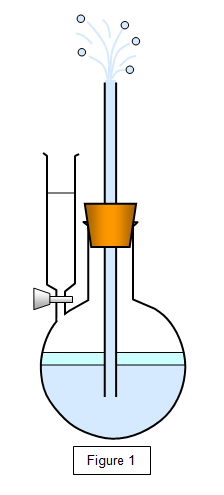You can show that the rate of evaporation may be increased by:
2. Increasing the area of the liquid surface.
3. Blowing a stream of air across the surface.
4. Reducing the pressure above the liquid surface.
Saturated vapours
When a liquid is in a closed container the space above the liquid is full of vapour, and the vapour is then described as a saturated vapour – this means that the density of the liquid molecules in the air is a maximum. This is due to molecules continually escaping and reentering the liquid. At any moment the number of molecules leaving the surface will be equal to the number returning to it and so a dynamic equilibrium is set up.
The properties of saturated vapours were first investigated by Dalton around 1800. This is shown in Figure 2(a), which shows a state before saturation has been reached (when there will be more molecules leaving the surface than returning to it) and Figure 2(b), which shows the saturated state. A dynamic equilibrium exits here.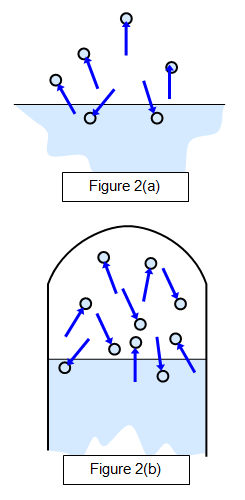This vapour will exert a pressure and if there is sufficient liquid the air above the liquid surface will be saturated with vapour; the pressure that this saturated vapour exerts is known as the saturated vapour pressure (s.v.p.) of the liquid at that temperature.
Notice that since the velocity of the molecules increases with temperature the saturated vapour pressure also increases with temperature, and therefore the temperature of the vapour must be specified when quoting its saturated vapour pressure (s.v.p.)
The Effect of Temperature on Saturated Vapour Pressure (S.V.P) of a Liquid
Explain the effect of temperature on saturated vapour pressure (S.V.P) of a liquid
Saturated vapour pressure (S.V.P):Is the pressure exerted by vapour when a liquid is heated and reaches a state of Equilibrium where eventually the rate at which the molecules leave the liquid is equal to the rate at which others return to it.
The Height, of mercury represents the saturated vapour pressure of the liquid in the flask. Saturated vapour pressure increases with the increase in Temperature (Ti) and the increased with decrease in Temperature (Td).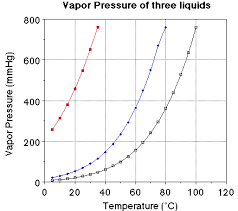The graph of saturated vapour pressure (svp) against Temperature
The graph shows as saturated vapour pressure (s.v.p) increases then the temperature will increase and vice versa for decrease of temperature.
Boiling point (B.P):Is the temperature reached where the saturated vapour pressure (S.V.P) is equal to external atmospheric pressure.
The Boiling point of alcohol is 78OC water is 100OC and pressure of the atmosphere as 76cm of mercury.The intersection of the normal atmosphere pressure line with the liquids S.V.P curve.
FORM THREE PHYSICS STUDY NOTES TOPIC TOPIC 7: MEASUREMENT OF THERMAL ENERGY & TOPIC 8: VAPOUR AND HUMIDITY
4/ 5
Oleh